# AP Macroeconomics : Real Interest Rate

## Example Questions

### Example Question #1 : Real Interest Rate

The real interest rate can be approximated by the Fisher equation: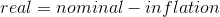What is the exact formula for the real interest rate?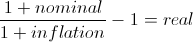The Fisher equation always gives the exact real interest rate.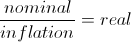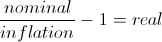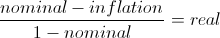Explanation:

The real interest rate is defined as the nominal appreciated value of assets divided by the new price level of the assets. The nominal appreciated value is simply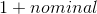, while the new price level is equal to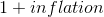. This gives the real appreciated value of assets. We then subtract 1 to get the real interest rate.

Example: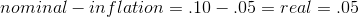(according to the Fisher equation)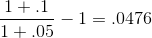The Fisher equation comes close, but is not actually correct.

### All AP Macroeconomics Resources